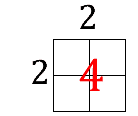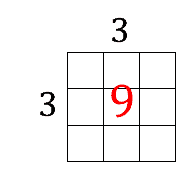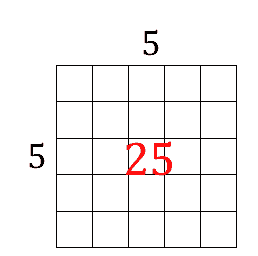# Mr. R.’s World of Math

## Square Numbers

### like 4, 9, and 25

4, and 9, and 25,
Laughed at 8, they felt real wise,
Square numbers rule, was their rant,
We graph as squares and you can’t!
You think you’re cool,
8 laughed out loud,
My factors rock,
They do me proud,
8 x 1,
4 x 2,
Not boring squares,
Like all of you!

Square numbers are the product of a number multiplied by itself.
An exponent (the small number after the base number) shows how many times to multiply a number by itself:
52 means multiply 5 x 5
53 means multiply 5 x 5 x 5
Square numbers can be graphed as a rectangular array with equal sides, or in other words, a square.
For example,

 2 X 2 = 4 3 X 3 = 9 5 X 5 = 25RELATED MATH CHALLENGES

If a right triangle has a side of 5 inches, and a hypotenuse of 13 inches, how long is the third side?

A runner runs diagonally across a football field that is 40 yards long and 30 yards wide, how far does the runner run?

A playground slide is 17 feet long, and travels 15 feet along the ground. What is the height of the ladder to the top of the slide? (assume all sides are straight)